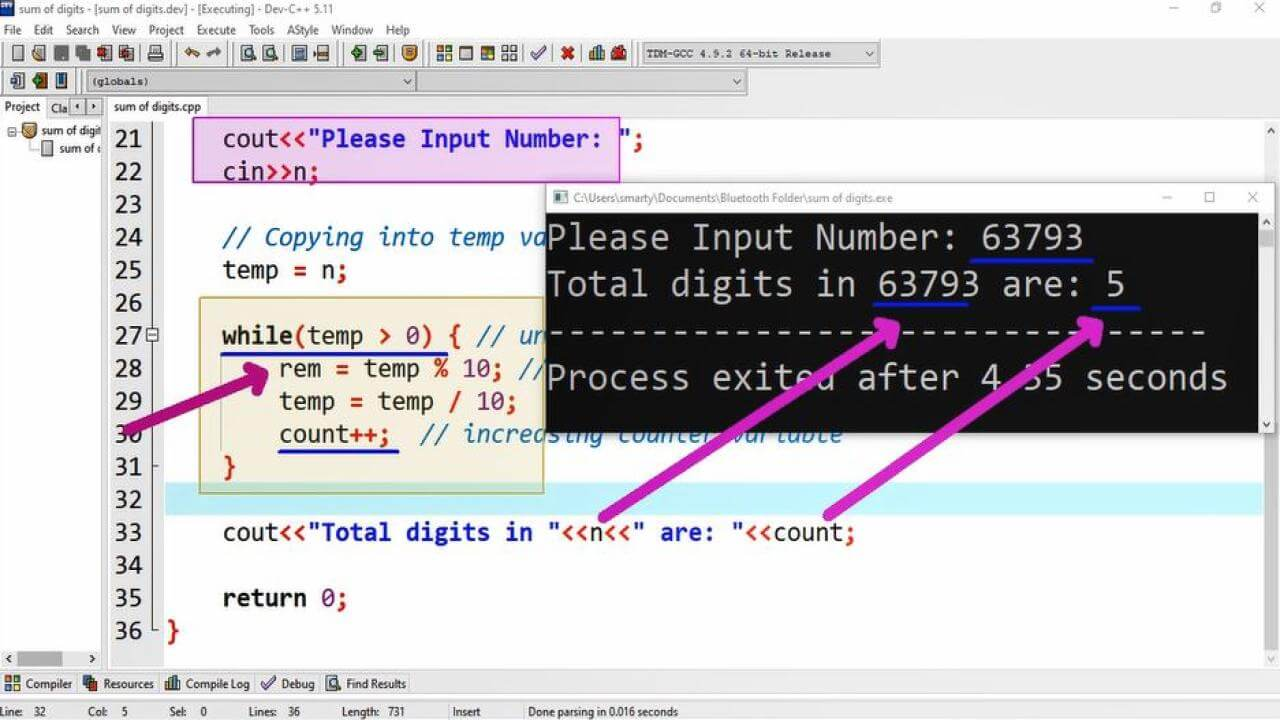# Count Digits Of Number Program Example using CPP

## Source Code:

#include <iostream>

using namespace std;

/*
Count No of Digits in Numbere
eg: if number N is 3421 Then
Digits of N = 4
*/

int main(int argc, char** argv) {

// variable declarations
int n;  // For input Number
int rem;  // Used to store remainder
int temp;  // Used to save copy of input

// Variable initialization
int count = 0; // Store No. of digits

cin>>n;

// Copying into temp variable
temp = n;

while(temp > 0) { // until all digits are get
rem = temp % 10; // calculating remainder
temp = temp / 10;  // reducing number
count++;  // increasing counter variable
}

cout<<"Total digits in "<<n<<" are: "<<count;

return 0;
}

## Output:## Working:

This program example use while loop for counting total digits of a given number. This number is taken in a variable of integer type. This variable is named as n. Here is the detail of variables declared in this program along with their description and use.

 Sr. Identifier Data Type Description 1 n int It is used to store user input 2 rem int rem variable is used to store in while loop for storing remainder value. 3 temp int temp variable will store additional copy of user input. 4 count int It will work similar to counter variable, that holds the total number of iterations.

First of all we declare all required variables such as n, temp and rem. Here we also initialize another variable named as count. This count variable will be updated in while loop. Each time when an iteration will run this counter variable will incremented by 1. At the end of iterations we will print count variable which is holding total no of digits.

Here we also declare an additional variable which is named as temp. This temp variable will hold copy of input variable. We will use this temp variable in while loop. After completion of while loop this temp variable will become 0. We can also implement this algorithm using do while loop or for loop as well.